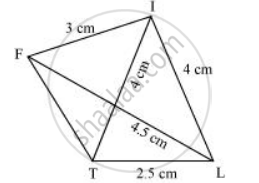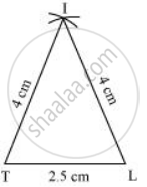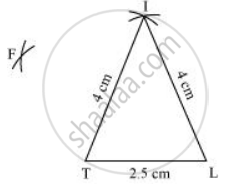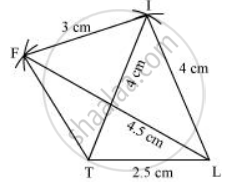Share

# Construct the Following Quadrilaterals. Quadrilateral Lift Li = 4 Cm If = 3 Cm Tl = 2.5 Cm Lf = 4.5 Cm It = 4 Cm - CBSE Class 8 - Mathematics

ConceptConstructing a Quadrilateral When Two Diagonals and Three Sides Are Given

LI = 4 cm

IF = 3 cm

TL = 2.5 cm

LF = 4.5 cm

IT = 4 cm

#### Solution

A rough sketch of this quadrilateral can be drawn as follows.1) Δ ITL can be constructed by using the given measurements as follows.2) Vertex F is 4.5 cm away from vertex L and 3 cm away from vertex I. Therefore, while taking L and I as centres, draw arcs of 4.5 cm radius and 3 cm radius respectively, which will be intersecting each other at point F3) Join F to T and F to I.